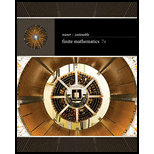# Encryption Matrices are commonly used to encrypt data. Here is a simple form such an encryption can take. First, we represent each letter in the alphabet by a number, so let us take &lt;space&gt; = 0 , A = 1 , B = 2 and so on. Thus, for example, “ABORT MISSION” becomes [ 1 2 15 18 20 0 13 9 19 19 9 15 14 ] To encrypt this coded phrase, we use an invertible matrix of any size with integer entries. For instance, let us take A to be the 2 × 2 matrix [ 1 2 3 4 ] . We can first arrange the coded sequence of numbers in the form of a matrix with two rows (using zero in the last place if we have an odd number of characters) and then multiply on the left by A: Encrypted matrix = [ 1 2 3 4 ] [ 1 15 20 13 19 9 14 2 18 0 9 19 15 0 ] = [ 5 51 20 31 57 39 14 11 117 60 75 133 87 42 ] Which we can also write as [ 5 11 51 117 20 60 31 75 57 133 39 87 14 42 ] To decipher the encoded message, multiply the encrypted matrix by A − 1 . Exercises 63–66 use the above matrix A for encoding and decoding. Decode the following message, which was encrypted using the matrix A . [ 33 69 54 126 11 27 20 60 29 59 65 149 41 87 ]### Finite Mathematics

7th Edition
Stefan Waner + 1 other
Publisher: Cengage Learning
ISBN: 9781337280426

#### Solutions

Chapter
Section### Finite Mathematics

7th Edition
Stefan Waner + 1 other
Publisher: Cengage Learning
ISBN: 9781337280426
Chapter 4.3, Problem 65E
Textbook Problem
2 views

## Encryption Matrices are commonly used to encrypt data. Here is a simple form such an encryption can take. First, we represent each letter in the alphabet by a number, so let us take <space> = 0 , A = 1 , B = 2 and so on. Thus, for example, “ABORT MISSION” becomes [ 1 2 15 18 20 0 13 9 19 19 9 15 14 ] To encrypt this coded phrase, we use an invertible matrix of any size with integer entries. For instance, let us take A to be the 2 × 2 matrix [ 1 2 3 4 ] . We can first arrange the coded sequence of numbers in the form of a matrix with two rows (using zero in the last place if we have an odd number of characters) and then multiply on the left by A: Encrypted matrix = [ 1 2 3 4 ] [ 1 15 20 13 19 9 14 2 18 0 9 19 15 0 ] = [ 5 51 20 31 57 39 14 11 117 60 75 133 87 42 ] Which we can also write as [ 5 11 51 117 20 60 31 75 57 133 39 87 14 42 ] To decipher the encoded message, multiply the encrypted matrix by A − 1 . Exercises 63–66 use the above matrix A for encoding and decoding.Decode the following message, which was encrypted using the matrix A. [ 33 69 54 126 11 27 20 60 29 59 65 149 41 87 ]

To determine

To calculate: The decoded form of the message  where each letter in the alphabet is represented by a number, and the sequence of numbers is arranged in the form of a matrix with two rows and the sequence is multiplied on the left by A where A=.

### Explanation of Solution

Given Information:

Each letter in the alphabet is represented by a number, and the sequence of numbers is arranged in the form of a matrix with two rows and the sequence is multiplied on the left by A where A=.

Formula used:

A matrix with m rows and n columns is of dimension m×n, where m and n are positive integers.

Calculation:

Consider the encoded message,



Write as a matrix with two rows as below,



To calculate the decoded message, multiply the inverse of the matrix A by the encoded message.

Thus, decoded message is 1.

Since, number of rows in 1 is 2 and number of columns is 2. Thus, dimension of 1 is 2×2.

Since, number of rows in  is 2 and number of columns is 7. Thus, dimension of  is 2×7.

Since, number of columns in 1 is equal to the number of rows in , the product 1 is defined

### Still sussing out bartleby?

Check out a sample textbook solution.

See a sample solution

#### The Solution to Your Study Problems

Bartleby provides explanations to thousands of textbook problems written by our experts, many with advanced degrees!

Get Started

Find more solutions based on key concepts
Fighting Crime Suppose the reported serious crimes (crimes that include homicide, rape, robbery, aggravated ass...

Applied Calculus for the Managerial, Life, and Social Sciences: A Brief Approach

Expand each expression in Exercises 122. (2x3)2

Finite Mathematics and Applied Calculus (MindTap Course List)

Write the sum in expanded form. 3. i=463i

Single Variable Calculus: Early Transcendentals

In Exercises 17 to 22, factor completely. 3y2+24y+45

Elementary Geometry for College Students